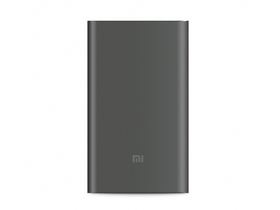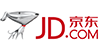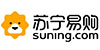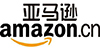# 小米 移动电源(10000mAh)高配版

USB Type-C接口，支持双向快充### 小米移动电源(10000mAh)高配版京东商城 小米（MI） 移动电源10000mAh大容量充电宝 手机平板通用 2代银色 ￥ 79.0

• 信用卡
• 自提
• 到付
• 网银
• 正品
• 发票
• 联保
• 7退15换
• 延保

• 信用卡
• 自提
• 到付
• 网银
• 正品
• 发票
• 联保
• 7退15换
• 延保

• 信用卡
• 自提
• 到付
• 网银
• 正品
• 发票
• 联保
• 7退15换
• 延保

txr521超薄青花瓷三星苹果小米手机通用充电宝 10000毫安聚合物移动电源 冲电宝 喜鹊闹枝 标准配置 ￥ 88.0

• 信用卡
• 自提
• 到付
• 网银
• 正品
• 发票
• 联保
• 7退15换
• 延保

txr533真皮超薄三星苹果小米手机通用充电宝 10000毫安聚合物移动电源 冲电宝 棕格纹 新品 标准配置 ￥ 88.0

• 信用卡
• 自提
• 到付
• 网银
• 正品
• 发票
• 联保
• 7退15换
• 延保

• 信用卡
• 自提
• 到付
• 网银
• 正品
• 发票
• 联保
• 7退15换
• 延保

txr521超薄青花瓷三星苹果小米手机通用充电宝 10000毫安聚合物移动电源 冲电宝 竹报平安 标配+充电头 ￥ 98.0

• 信用卡
• 自提
• 到付
• 网银
• 正品
• 发票
• 联保
• 7退15换
• 延保

txr523超薄青花瓷三星苹果小米手机通用充电宝 10000毫安聚合物移动电源 冲电宝 游龙戏凤 标配+充电头 ￥ 98.0

• 信用卡
• 自提
• 到付
• 网银
• 正品
• 发票
• 联保
• 7退15换
• 延保

• 信用卡
• 自提
• 到付
• 网银
• 正品
• 发票
• 联保
• 7退15换
• 延保

txr529真皮超薄三星苹果小米手机通用充电宝 10000毫安聚合物移动电源 冲电宝 棕格纹 新品 标配+充电头 ￥ 98.0

• 信用卡
• 自提
• 到付
• 网银
• 正品
• 发票
• 联保
• 7退15换
• 延保

txr533真皮超薄三星苹果小米手机通用充电宝 10000毫安聚合物移动电源 冲电宝 棕格纹 新品 标配+充电头 ￥ 98.0

• 信用卡
• 自提
• 到付
• 网银
• 正品
• 发票
• 联保
• 7退15换
• 延保

txr531真皮超薄三星苹果小米手机通用充电宝 10000毫安聚合物移动电源 冲电宝 玫瑰红 标配+充电头 ￥ 98.0

• 信用卡
• 自提
• 到付
• 网银
• 正品
• 发票
• 联保
• 7退15换
• 延保

• 信用卡
• 自提
• 到付
• 网银
• 正品
• 发票
• 联保
• 7退15换
• 延保

• 信用卡
• 自提
• 到付
• 网银
• 正品
• 发票
• 联保
• 7退15换
• 延保

txr532真皮超薄三星苹果小米手机通用充电宝 10000毫安聚合物移动电源 冲电宝 棕格纹 新品 标配+充电头 ￥ 98.0

• 信用卡
• 自提
• 到付
• 网银
• 正品
• 发票
• 联保
• 7退15换
• 延保

• 信用卡
• 自提
• 到付
• 网银
• 正品
• 发票
• 联保
• 7退15换
• 延保

• 信用卡
• 自提
• 到付
• 网银
• 正品
• 发票
• 联保
• 7退15换
• 延保

• 信用卡
• 自提
• 到付
• 网银
• 正品
• 发票
• 联保
• 7退15换
• 延保

• 信用卡
• 自提
• 到付
• 网银
• 正品
• 发票
• 联保
• 7退15换
• 延保

• 信用卡
• 自提
• 到付
• 网银
• 正品
• 发票
• 联保
• 7退15换
• 延保

• 信用卡
• 自提
• 到付
• 网银
• 正品
• 发票
• 联保
• 7退15换
• 延保

• 信用卡
• 自提
• 到付
• 网银
• 正品
• 发票
• 联保
• 7退15换
• 延保苏宁易购 小米（MI）移动电源高配版（10000mAh）灰色 轻薄便携充电宝 双向快充 高密度锂聚合物电芯 仅12.58mm薄 ￥ 149.0

• 信用卡
• 自提
• 到付
• 网银
• 正品
• 发票
• 联保
• 7退15换
• 延保

• 信用卡
• 自提
• 到付
• 网银
• 正品
• 发票
• 联保
• 7退15换
• 延保

• 信用卡
• 自提
• 到付
• 网银
• 正品
• 发票
• 联保
• 7退15换
• 延保

• 信用卡
• 自提
• 到付
• 网银
• 正品
• 发票
• 联保
• 7退15换
• 延保

• 信用卡
• 自提
• 到付
• 网银
• 正品
• 发票
• 联保
• 7退15换
• 延保亚马逊 小米（MI）小米新款移动电源/充电宝10000mAh (红色) ￥ 88.0

• 信用卡
• 到付
• 网银
• 正品
• 发票
• 联保

• 信用卡
• 到付
• 网银
• 正品
• 发票
• 联保

• 信用卡
• 到付
• 网银
• 正品
• 发票
• 联保